## DC motor

The function of a motor is to convert electrical energy into mechanical energy. From the following picture, we can see that a DC motor includes two round dots and one square dot. The round dots are used to connect with mechanical components, and the square dot with electrical components.

In CASPOC, the main difference between electrical and mechanical components is the surrounding dots of a component for connecting with others. The round dots to electrical components pass voltage or current signals. The square dots to mechanical components pass the mechanical signals which cause by mechanical motions, such as angular velocity. Hence we can see that a motor are an important interface between electrical and mechanical components.

According to the characteristics of electrical energy, we can use a DC or AC motor to connect with the voltage source which provides a certain current level to drive the rotor of a motor. The higher current we can have from the voltage source, the higher angular speed we can reach in a motor. Therefore, the minimum current to drive a motor will depend on the inertias of mechanical loads and the bearings to these objects. A bearing is used to make sure that a rotating object will rotate in the target position but it also results in a friction which resists the rotation. In previous example, we have done several models made by electrical components. In this example, we will show how to use these mechanical components by adding a simple DC motor in CASPOC.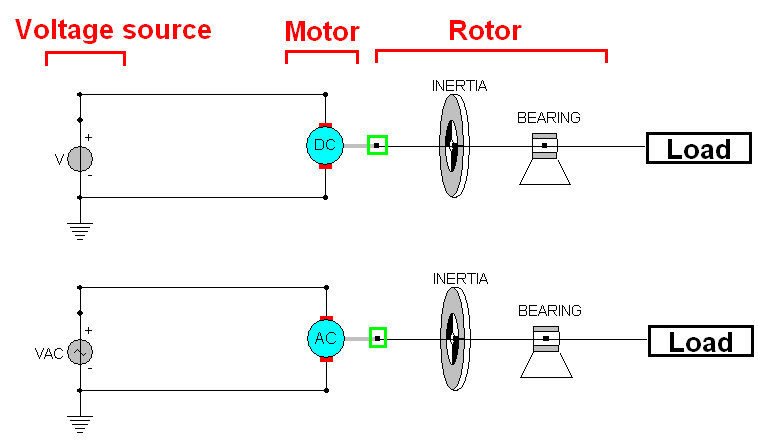The transformation from the electrical energy to the mechanical energy can be estimated by the ratio of the mechanical power and the electrical power. The electrical power can be calculated by the product of the current and the voltage. The mechanical power can be calculated by the product of the torque and the angular velocity.

Left-click Components/Circuit/Sources (step 1) and release the mouse. The DC voltage source follows the cursor and right-click to change its direction (step 2). Until the anode is upward, left-click on the workscreen to put the DC voltage source (step 3). Currently we have not set an electric ground so that CASPOC will give a suggested message to insert an electric ground automatically. Click yes to insert the ground (step 4).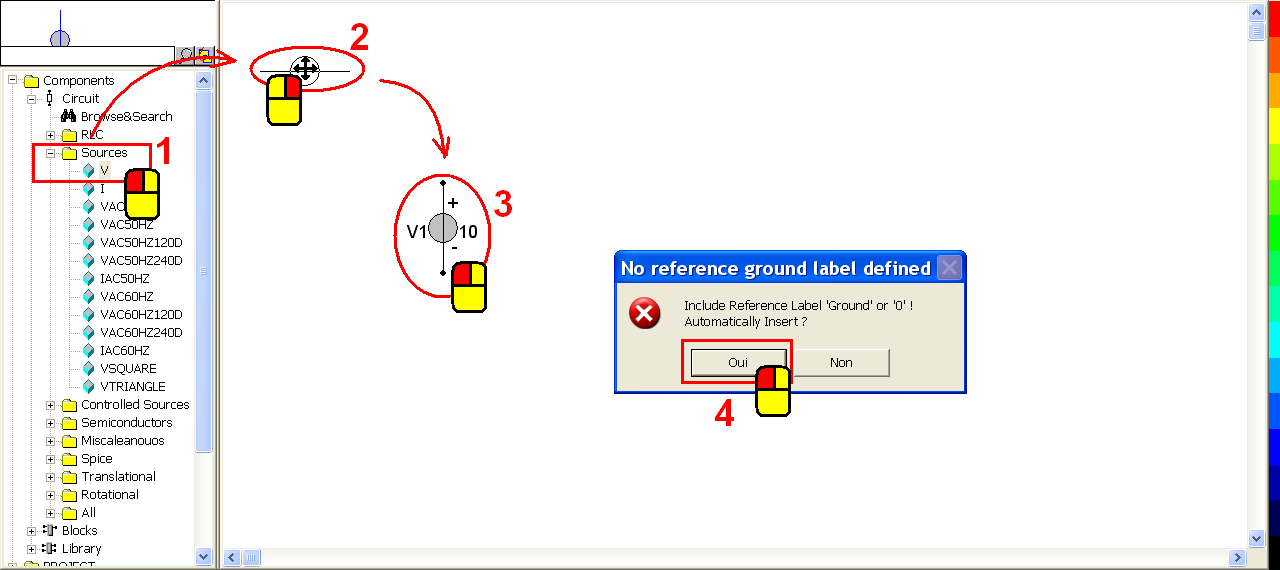Make sure there is a ground label shown in the anode of the DC voltage source after the auto-insertion (step 1). To receive the DC current from the DC voltage source, we choose a DC motor from the component list. Left-click Components/Library/ElectricalMachines/DC/pmdc (step 2) and release the mouse. The DC motor follows the cursor. If the angular velocity output (square dot) isn�t rightward, right-click to change its direction. Left-click to put the DC motor on the workscreen. Now we can see the surrounding dots of the DC motor: two round dots stand for the anode and cathode of the DC motor, one square dot sends the angular speed to other mechanical components (shown in green). Right-click on the DC motor (step 3) to see its parameters. In the parameter block diagram, we can see several parameters (step 4). To know the details of these parameters, click �Help Lib� (step 5) to see the explanation:

• Kt [Nm/A] = Ke[V/rad/s]=PMflux[Weber]=0.256 (Machine constant)
• Inertia [Kg m�] = 2m (Rotor inertia)
• LA = 5m (Stator inductance)
• Ra = 0.07 (Stator resistance)
• Friction [Nm/Rad/s] = 1u (Linear friction due to bearing/windage)

Click OK to close the pop-window (step 6).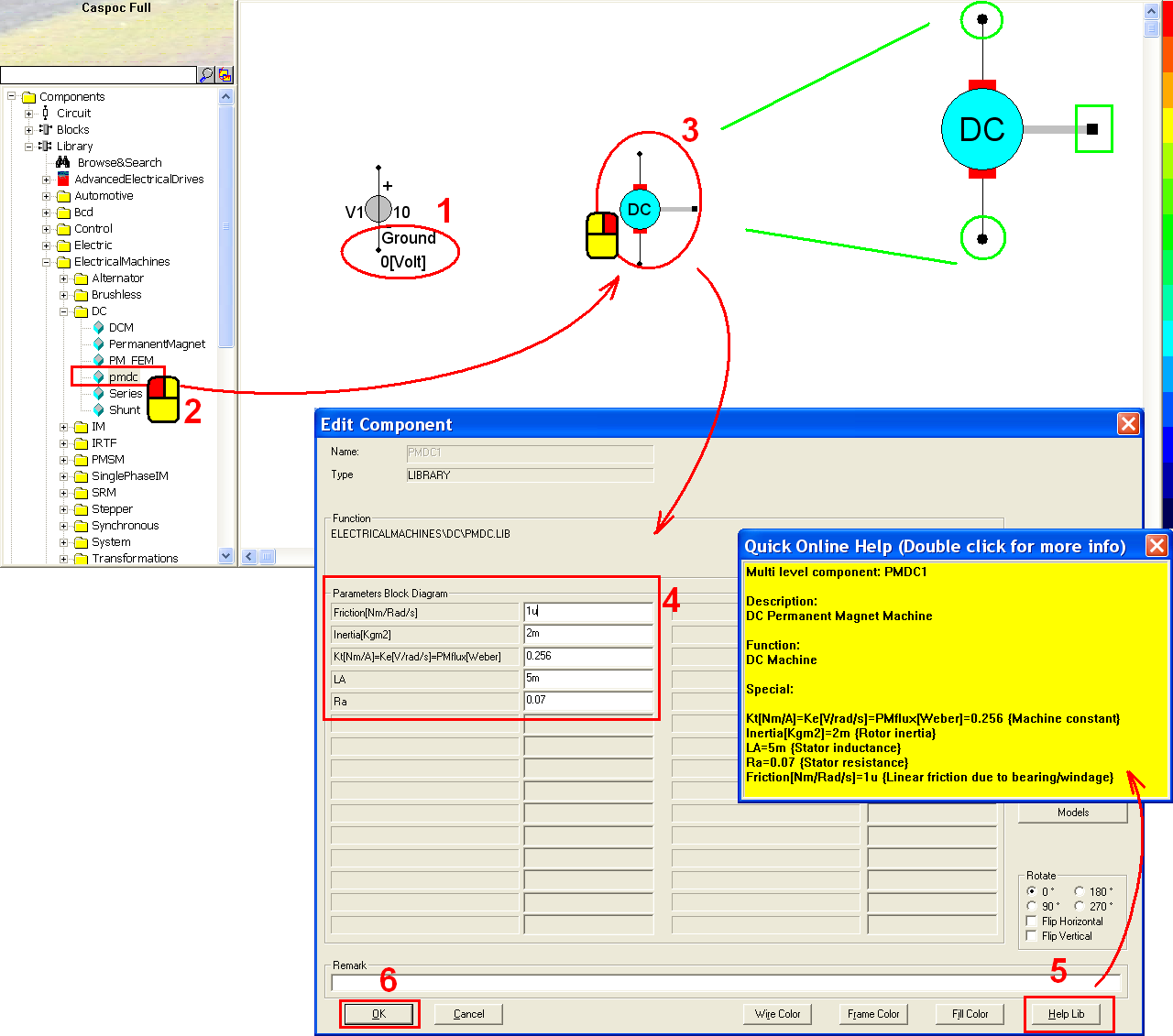If the voltage level to the DC motor changes, its output torque will change too. To control the angular speed of the DC motor, we can simply use a resistor. A higher resistance can drop the current value in the electrical loop and lower the voltage level to the DC motor, so that the angular velocity will also be dropped. Left-click Components/Circuit/RLC/R (step 1) and release the mouse. The resistor follows the cursor and right-click to change its direction (step 2). While the anode of the resistor is rightward (see the little dot around it), left-click on the workscreen to put it between the DC voltage source and the DC motor. Right-click the resistor (step 3) to change the resistance value to 1m (step 4). Click OK to close this pop-window (step 5).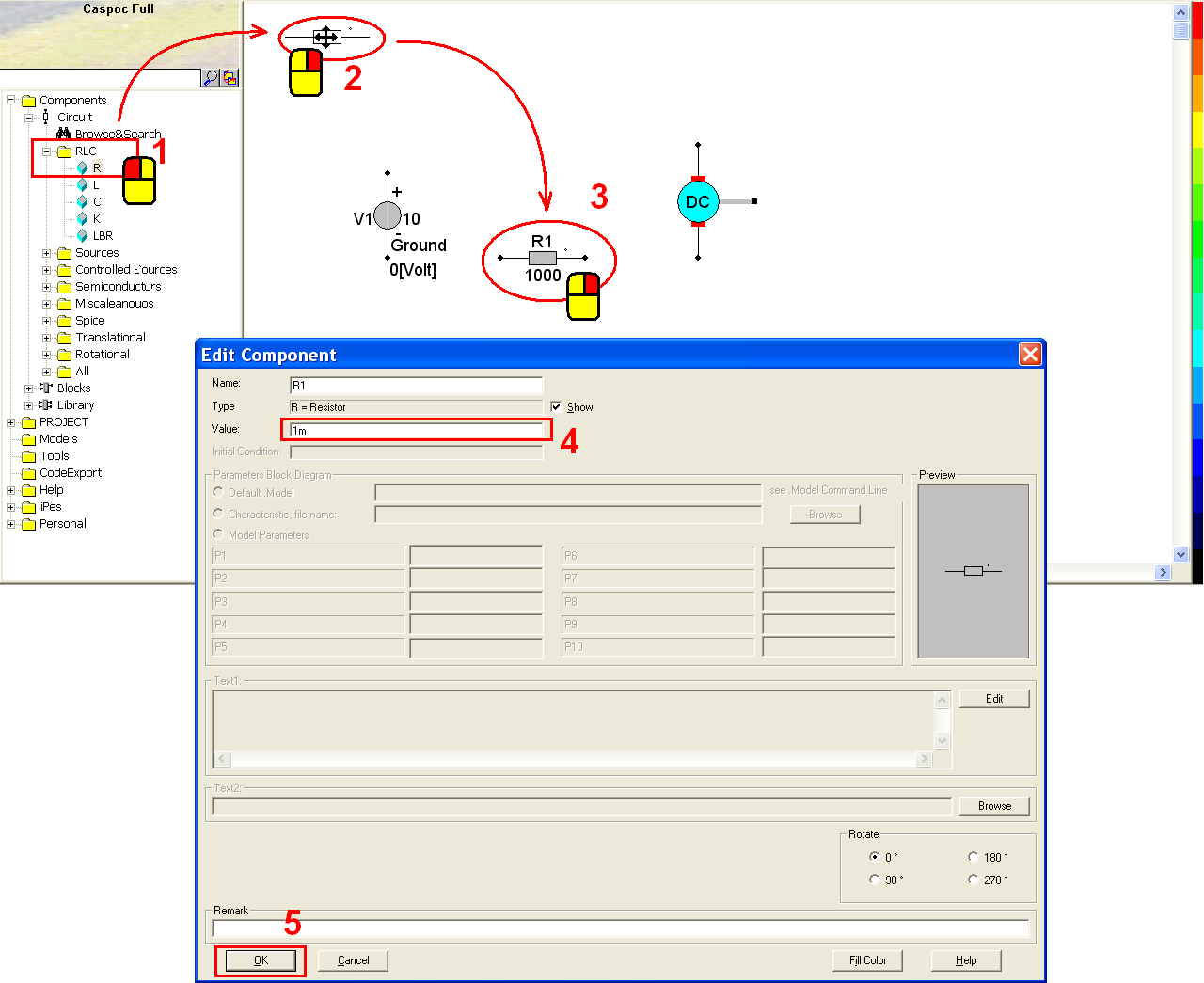To control the torque of a motor, we can also use other control methods, such as the PWM on/off control. We will show that in the next example.

Connect the DC voltage source, the DC motor and the resistor with the following configuration (step 1). Then the electrical part is finished. Now we can start to build the mechanical part from the output of the DC motor namely angular velocity. According to the angular velocity, we choose to use rotational components. If the DC motor rotates simply a mechanical load, we will consider its bearing and inertia which stand for the friction and the object mass respectively. Left-click Components/Circuit/Rotational/INERTIA (step 2) and release the mouse. The inertia follows the cursor and right-click to change its direction (step 3). Until the inertia is upward, left-click on the workscreen to put it in the right side of the DC motor. Right-click the inertia (step 4) to change its parameter. Change the value to 100m (step 5) and click OK to save the setting (step 6).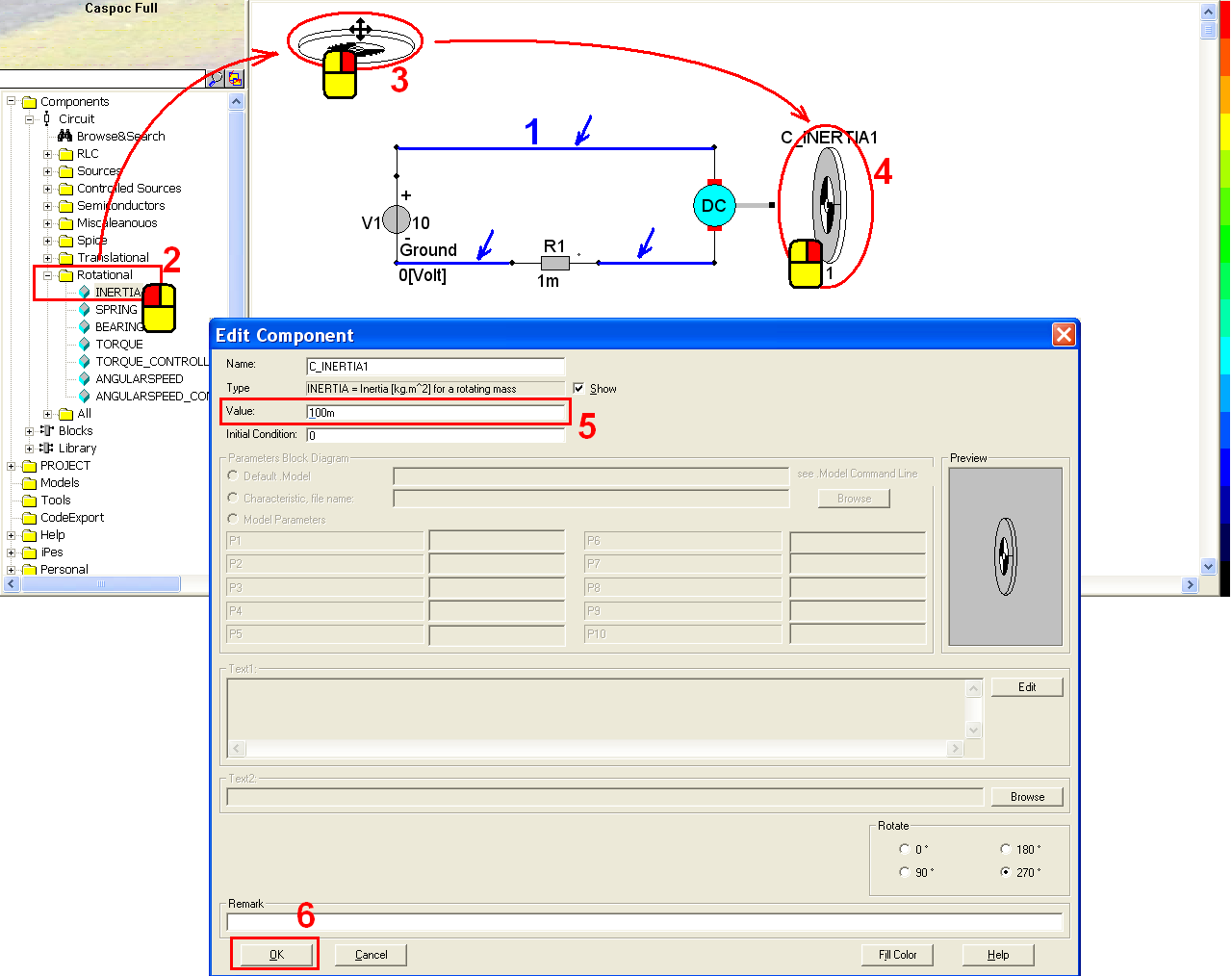Similarly, left-click Components/Circuit/Rotational/BEARING (step 1) and release the mouse. The bearing follows the cursor and right-click to change its direction (step 2). Left-click on the workscreen to put the bearing in the right side of the inertia. Right-click on the bearing (step 3) to change its parameter. Change the value to 200m (step 4) and click OK to save the setting (step 5).Connect all the mechanical components with the following configuration (step 1). Then we use a scope to observe the angular velocity of the load. Click the scope icon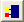in the experience bar (step 2) to put a scope in the right side of the bearing in order to observe the simulation result. Left-click the right-bottom corner of the scope and hold down the mouse to enlarge this scope (step 3).Connect the bearing to the first blue input trace (step 1). Click the scope iconin the experience bar (step 2) to put another scope below the resistor. Left-click the right-bottom corner of the scope and hold down the mouse to enlarge this scope (step 3).Right-click the first blue input trace of the second scope and enter Trace=Current through:R1 in the pop-window.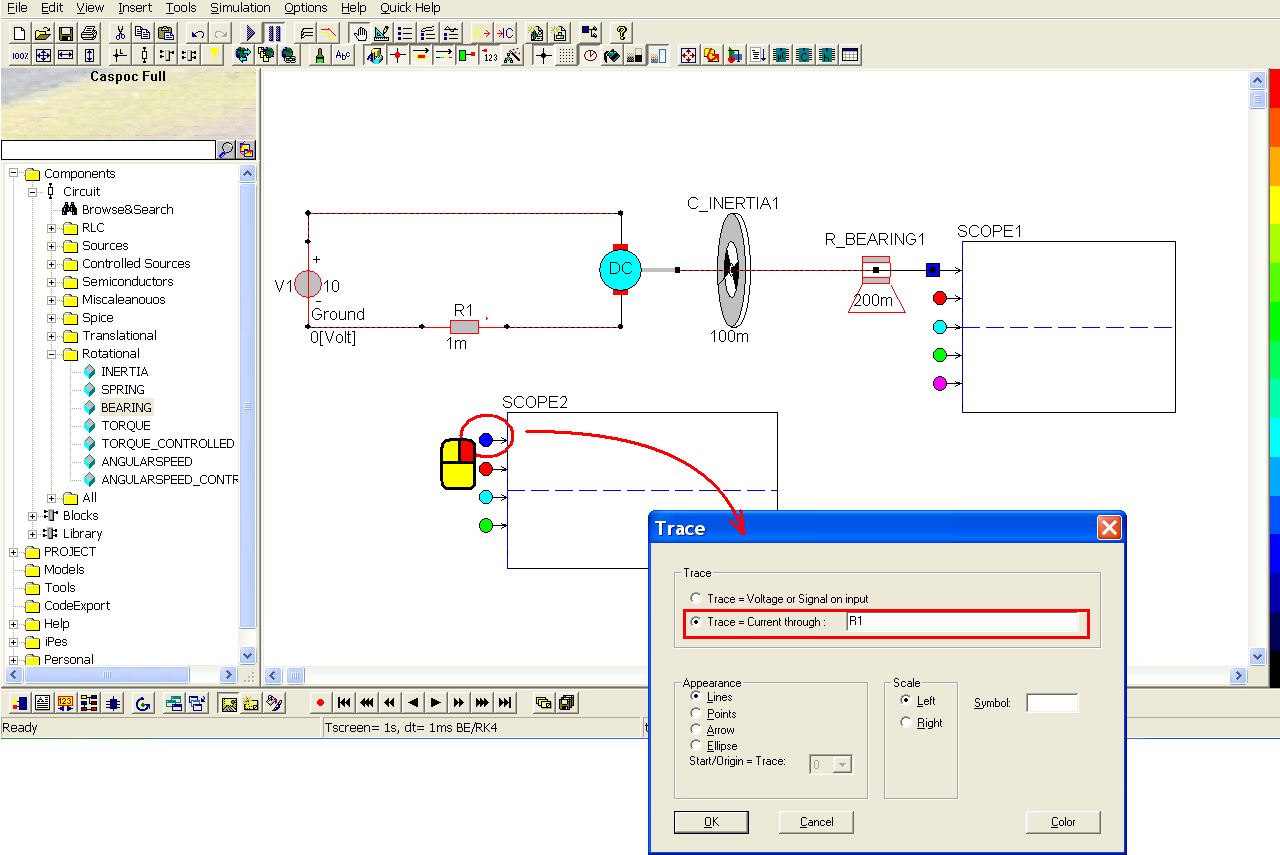Click the short-cut of simulation parameter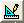(step 1). In the pop-window, select Euler for the Numerical Integration Method (step 2), Tscreen = 1, dt = 1m (step 3) and then click OK to save the setting.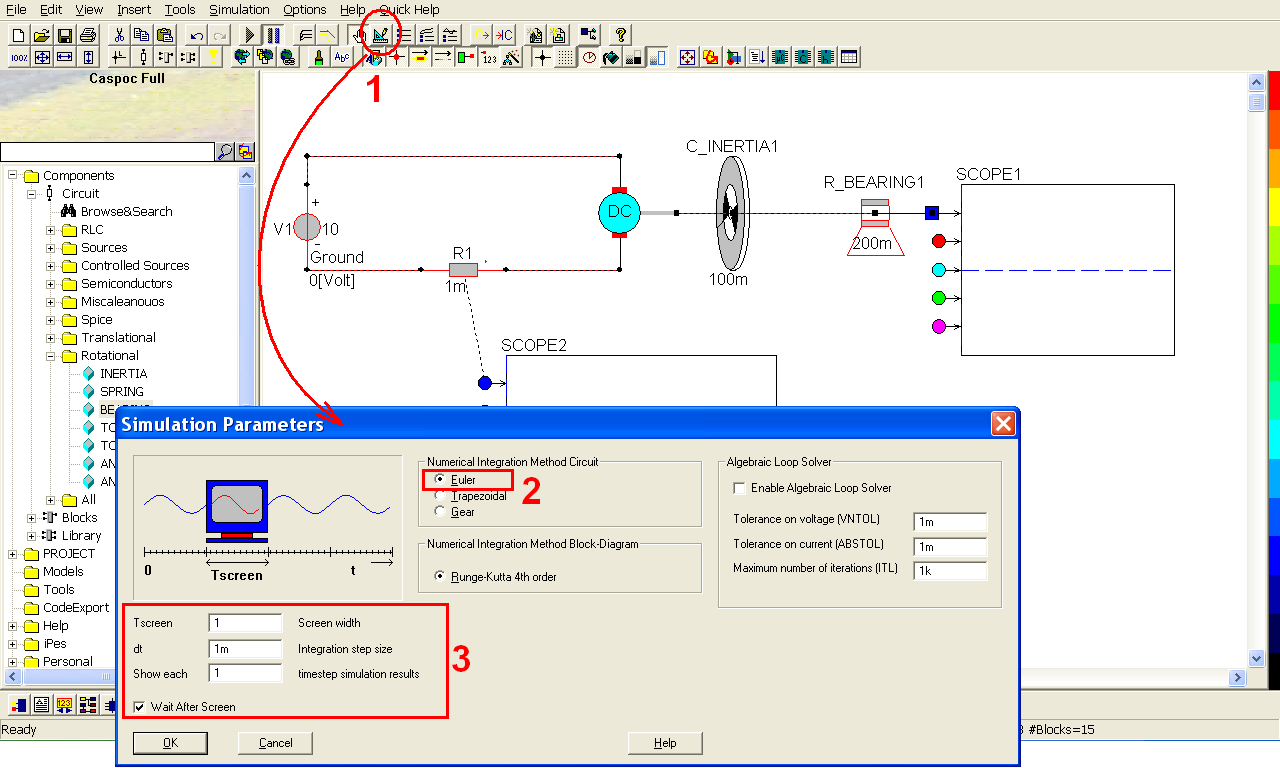Click the short-cut of start simulation(step 1). Right-click on the first scope (step 2) to see the details of the simulation result (step 3).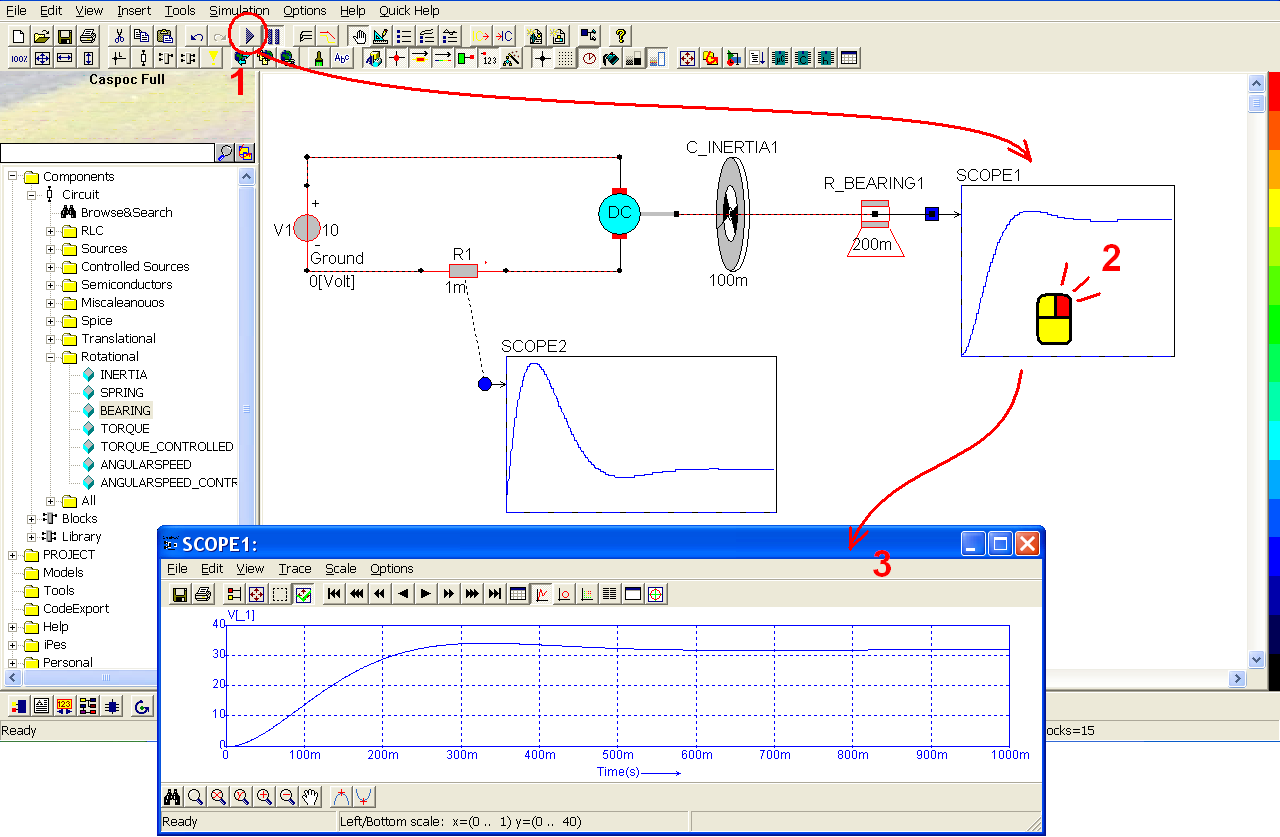Left-click and hold down the mouse to select the inertia, the bearing and the first scope (step 1). Release the mouse bottom after the selection. Left-click and hold down the mouse to move the three components to the right side at the same time (step 2). Notice to keep some space between the DC motor and the inertia.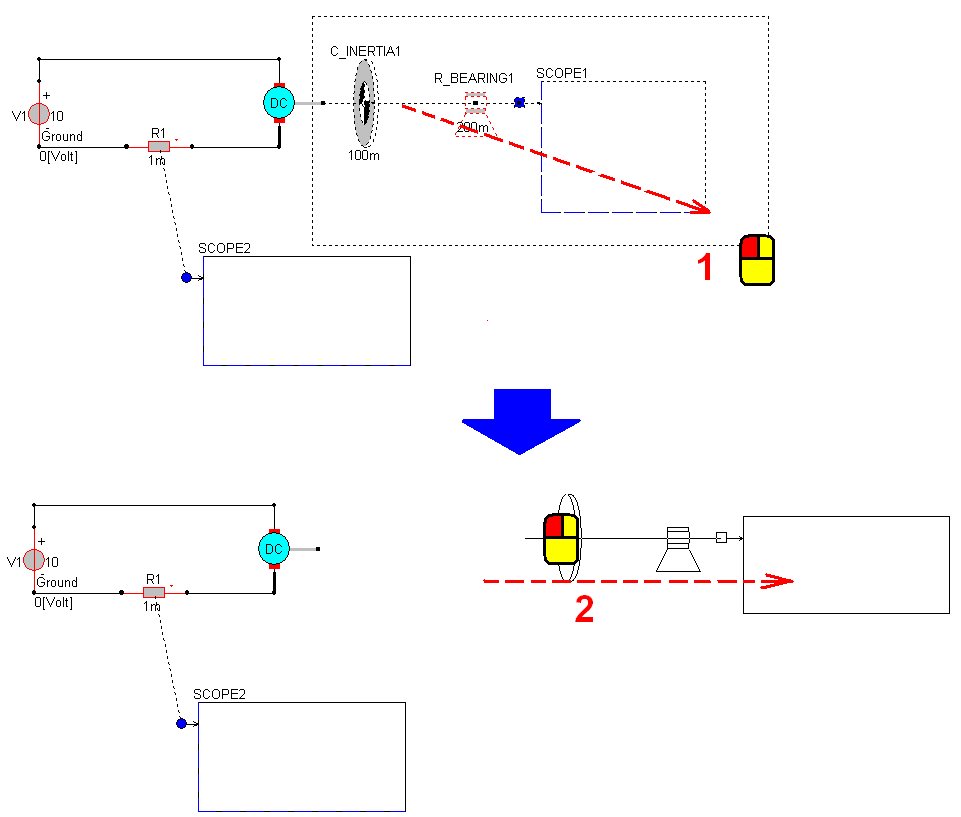In order to measure some information of the rotating object namely the mechanical load, such as the angular velocity, the torque and the power, we put a sensor between the motor and the inertia. Left-click Components/Library/Sensor/Rotational/PowerTorque (step 1) and release the mouse. The power torque sensor follows the cursor and right-click to change its direction if necessary. While the four outputs are upward, left-click to put it in the right side of DC motor to read the information from the motor (step 3). Connect the sensor and the inertia if they�re not (step 4).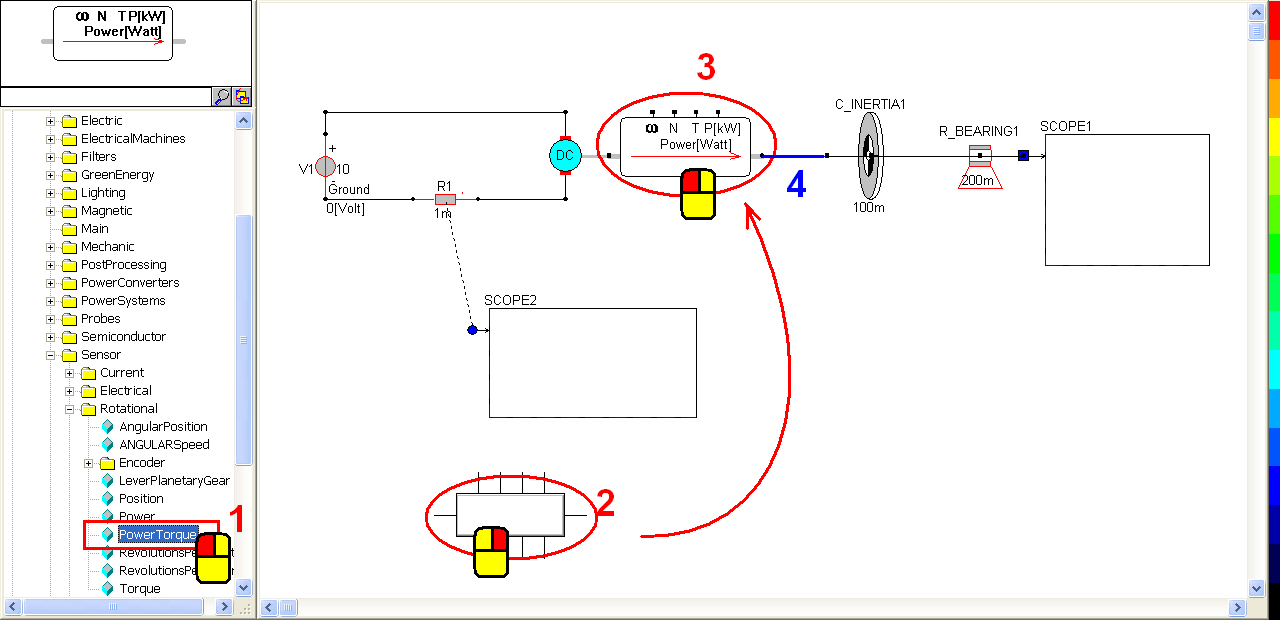To see the measured information, we can simply use a scope. Click the scope iconin the experience bar (step 1) to put one more scope above the inertia (step 2). Connect the four outputs from the power torque sensor to the four input trace of the third scope (step 3).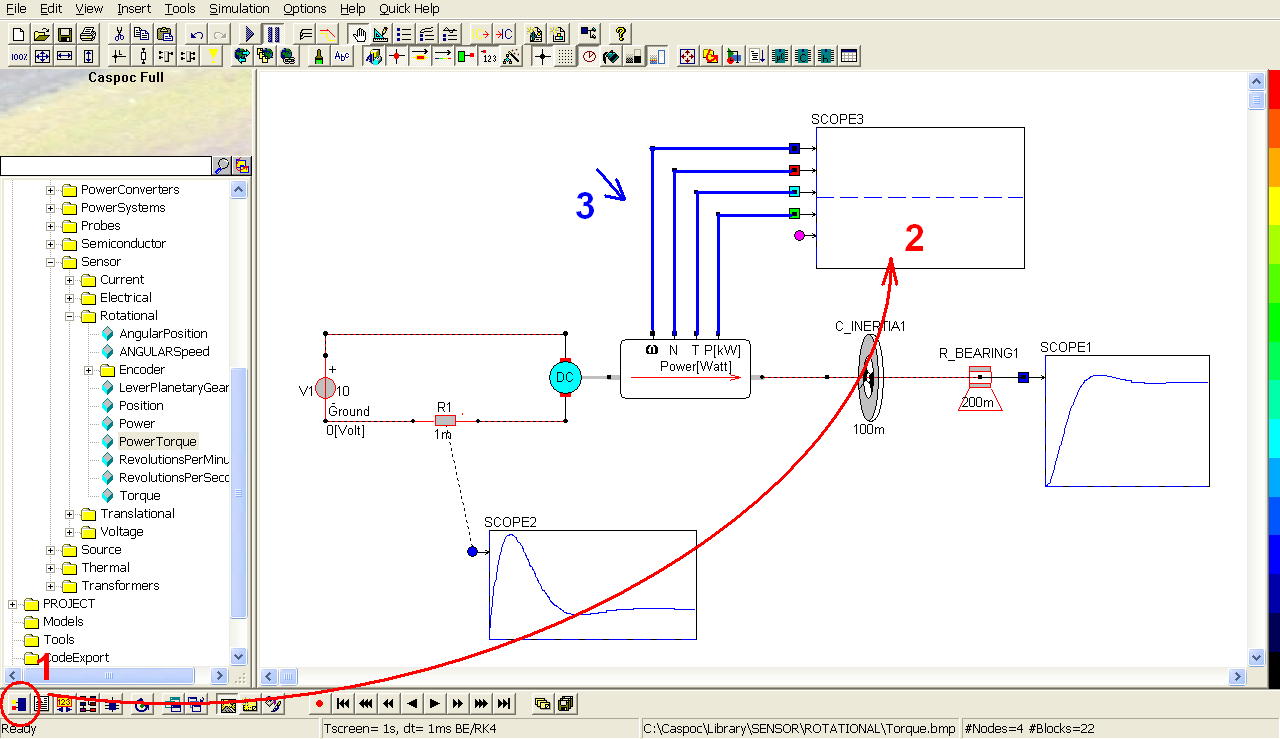Click the short-cut of start simulation(step 1) and then we can see the measured sensor information is shown in the third scope (step 2). Also the information can be saved in a txt file. Refer to Introduction/ Scope about the use of scope.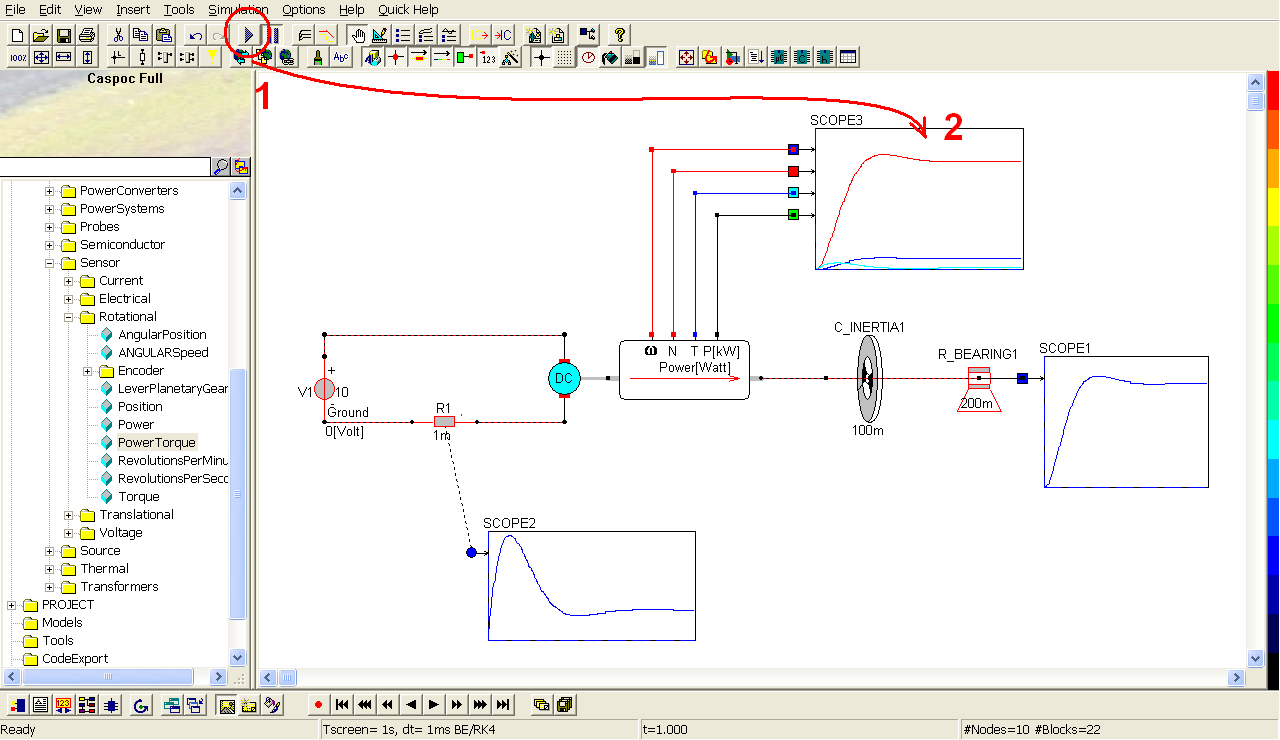To calculate the electrical energy, right-click the second scope and get the following window. Click the shortcut of listbox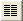(step 1) to enable the numeric display of simulation result. If we want to know the current value at 1 second, click Right or Left key to find it while t = 1 (step 2). In the listbox, we can see that the current value is 25.088 A at 1 second (step 3). Hence the electrical power equals to the product of the voltage and the current value, that is, 10 volt * 25.088 A = 250.88 watt.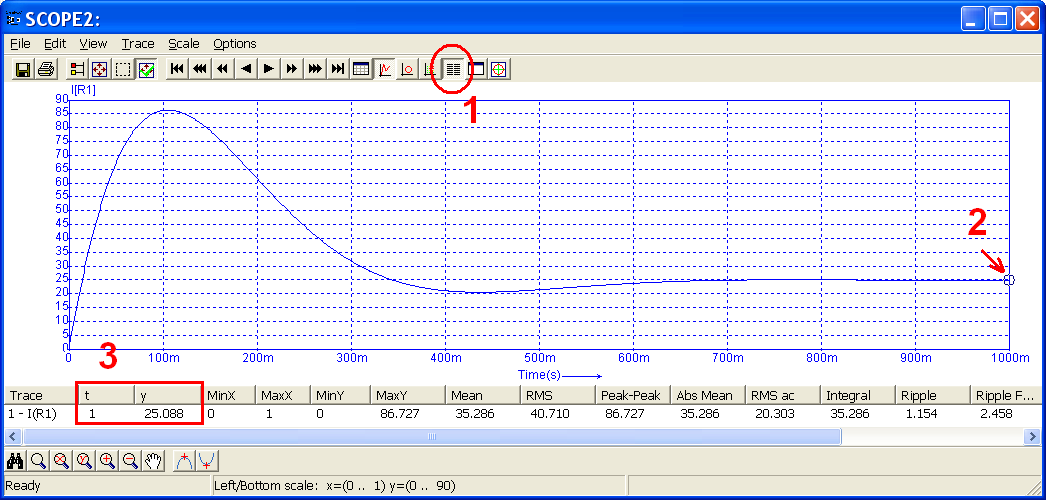To calculate the mechanical energy, right-click the third scope and get the following window. Click the shortcut of listbox(step 1) to enable the numeric display of simulation result. If we want to know the torque and angular speed at 1 second, click Right or Left key to find it while t = 1 (step 2). In the listbox, we can see that the angular speed is 32.116 rad/s at 1 second and the torque is 6.423 Nm (step 3). Hence the mechanical power equals to the product of the angular speed and the torque, that is, 32.116 * 6.423 = 206.281 ~ 206.272 watts.Power loss = electrical power � mechanical power = 250.88 - 206.272 = 44.608

The power loss is caused by the resistance of winding in the DC motor = I�R = 25.088 * 25.088 * 0.07 = 44.058